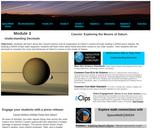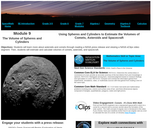Updating search results...

# 26 Results

View
Selected filters:
• Space MathRating
0.0 stars

This is a booklet containing 31 problem sets that involve a variety of math skills, including scientific notation, simple algebra, and calculus. Each set of problems is contained on one page. Learners will use mathematics to explore varied space science topics including black holes, ice on Mercury, a mathematical model of the Sun's interior, sunspots, the heliopause, and coronal mass ejections, among many others.

Subject:
Science
Material Type:
Lesson
Provider:
NASA
Provider Set:
Space Math
07/31/2019Rating
0.0 stars

This collection of activities is based on a weekly series of space science mathematics problems distributed during the 2012-2013 school year. They were intended for students looking for additional challenges in the math and physical science curriculum in grades 5 through 12. The problems were created to be authentic glimpses of modern science and engineering issues, often involving actual research data. The problems were designed to be one-pagers with a Teacher’s Guide and Answer Key as a second page.

Subject:
Science
Material Type:
Lesson
Provider:
NASA
Provider Set:
Space Math
07/31/2019Rating
0.0 stars

This is a booklet containing 87 problem sets that involve a variety of math skills, including scale, geometry, graph analysis, fractions, unit conversions, scientific notation, simple algebra, and calculus. Each set of problems is contained on one page. Learners will use mathematics to explore varied space science topics in the areas of Earth science, planetary science, and astrophysics, among many others. This booklet can be found on the Space Math@NASA website.

Subject:
Science
Material Type:
Lesson
Provider:
NASA
Provider Set:
Space Math
07/31/2019Rating
0.0 stars

This book presents 49 space-related math problems published weekly on the SpaceMath@NASA site during the 2011-2012 academic year. The problems utilize information, imagery, and data from various NASA spacecraft missions that span a variety of math skills in pre-algebra and algebra.

Subject:
Science
Material Type:
Lesson
Provider:
NASA
Provider Set:
Space Math
07/31/2019Rating
0.0 stars

This is a set of three, one-page problems about the sizes of moons in the solar system. Learners will use decimals to compare the sizes and distances of Saturn's moons to the center of Saturn. Options are presented so that students may learn about the Cassini mission through a NASA press release or by viewing a NASA eClips video [4 min.] about these and other moons in our solar system. This activity is part of the Space Math multi-media modules that integrate NASA press releases, NASA archival video, and mathematics problems targeted at specific math standards commonly encountered in middle school.

Subject:
Science
Material Type:
Lesson
Provider:
NASA
Provider Set:
Space Math
07/31/2019Rating
0.0 stars

This is a set of three, one-page problems about calculating the volume of objects. Learners may calculate the volume of an asteroid, Vesta, or the stacking of satellites inside an atlas V rocket nose cone. Options are presented so that students may learn about the Dawn mission to asteroid Vesta through a NASA press release or about NASA's investigation of comets by viewing a NASA eClips video [5 min.]. This activity is part of the Space Math multi-media modules that integrate NASA press releases, NASA archival video, and mathematics problems targeted at specific math standards commonly encountered in middle school.

Subject:
Science
Material Type:
Lesson
Provider:
NASA
Provider Set:
Space Math